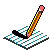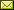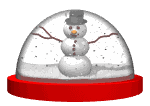PHYSICS@HORTONPhysics12

Physics 11

Math 11

NS Exams

Curriculum

Tips

Tutorials

McGraw Hill

Resources

Need it?

News

Enrichment

GiancoliIB Physics SL

April 2022 Notes

 April 1 Projectile Motion Penny Demo Projectile launched and dropped at same time Shoot-n-Drop Video Analysis of Projectile Motion (Ball Toss ~ Physics Tool Kit) Video Analysis of Projectile Motion (Bird Toss in moving chair ~ Logger Pro) Notes April 4STORM DAY April 5 Projectile Practice from McGraw-Hill (see post in Google Classroom) Notes April 6 WORK CLASS You need lots of practice: Calculator Pad work on 2-1 TO DO List Acceleration Simulation (Wiley) Graphing Motion (Shockwave animation ~ use Internet Explorer or Firefox) OR Graphs & Ramps (for Chromebooks & mobile devices) April 7 Error Analysis Lab Motion on an Incline (not this year) April 8 PRESCRIBED PRACTICAL ~ Measuring the acceleration due to gravity by timing a freefalling object (write up as DCP/CE) Use the following simulation: Free Fall Model April 10 April 11 QUIZ (2.1 Kinematics) Investigating the Fall of a Coffee Filter (Design) work on Labs April 12 Forces (Weight/Normal Force/FBDs) BrainPopUK: Gravity What is Gravity? What is a Force? What Forces are Acting on You? Forces and Motion Simulation Lab Notes April 13 Forces (Resultant Forces/Balanced & Unbalanced Forces/Newton's First Law/Translational Equilibrium/Newton's Second Law) Notes April 14 Forces & Motion Simulation Lab (Acceleration Module) Finish "Popper Physics" Finish "Error Analysis" and "Freefall" Work on "TO DO" list April 19 Forces (Newton's Second Law) Brain PopUK: Force Negative Mass?? Notes April 20 Forces (Friction) Forces (Fluid Resistance) Forces in 1D (needs Java) The Physics Classroom The Calculator Pad Notes April 21 Investigating the Terminal Velocity of a Coffee Filter April 22 Work on Terminal Velocity Lab April 25 Momentum & Impulse (Momentum, Impulse & Newton's Second Law/F-t graphs/Conservation of Linear Momentum) Momentum & Impulse More Momentum & Impulse Impulse Bouncing Ball Momentum & Collisions (PHYSCLIPS) What is wrong in the NS Driver's Handbook? (see p51) Notes April 26 Momentum & Impulse (Conservation of Linear Momentum) pHET Collision Lab (settings: 1D, check velocity vectors & momenta diagram, try 100%, 50%, 0% elasticity, try different mass combinations, try different velocities and look at the total mometum before & after collision) 2D Collisions (World-in-Motion video analysis) April 27 Momentum & Impulse (Conservation of Linear Momentum) Cannon Ball Fired from Back of Moving Truck Recoil Velocity Animation Notes1 Notes2 Momentum & Impulse (Conservation of Linear Momentum) Notes Video: Momentum (Vector Addition) ~ see Google Classroom April 29 Finish up lab work: Terminal Velocity Lab pHET Collision Lab 2D Collisions (World-in-Motion video analysis)

{subdirectory}